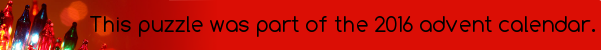mscroggs.co.uk
mscroggs.co.uksubscribe

# Puzzles

## Is it equilateral?

In the diagram below, $$ABDC$$ is a square. Angles $$ACE$$ and $$BDE$$ are both 75°.
Is triangle $$ABE$$ equilateral? Why/why not?

## 17 DecemberThe number of degrees in one internal angle of a regular polygon with 360 sides.

## Ticking clock

Is there a time of day when the hands of an analogue clock (one with a second hand that moves every second instead of moving continuously) will all be 120° apart?
Tags: angles, time

## Dodexagon

In the diagram, B, A, C, D, E, F, G, H, I, J, K and L are the vertices of a regular dodecagon and B, A, M, N, O and P are the vertices of a regular hexagon.
Show that A, M and E lie on a straight line.

## Three squares

Source: Numberphile
The diagram shows three squares with diagonals drawn on and three angles labelled.
What is the value of $$\alpha+\beta+\gamma$$?

## Archive

Show me a random puzzle
▼ show ▼
© Matthew Scroggs 2012–2021